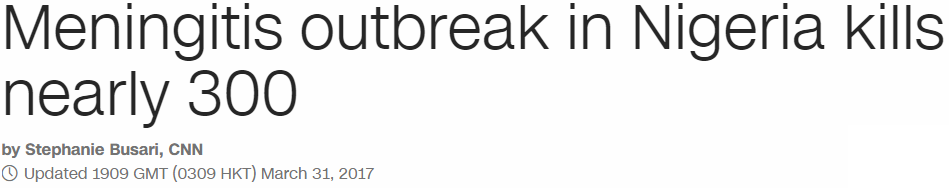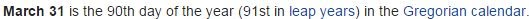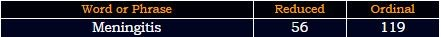## Cipher## Apr 1, 2017

### CNN - Meningitis outbreak in Nigeria kills nearly 300 + Zika vaccine

Yesterday was March 31st, a date with 44 numerology and we see a story from CNN about nearly 300 people killed due to Meningitis outbreak

3/31/2017 = 3+31+2+0+1+7 = 44
Disease = 4+9+10+5+1+10+5 = 44 (S Exception)
Kill = 11+9+12+12 = 44 (Ordinal)
3/31/2017 = 3+3+1+2+0+1+7 = 17 - "seventeen" = 8+4+5+4+4+7+4+4+4 = 44 (Reverse Reduced)

3/31/17 = 3+31+17 = 51 - "outbreak" = 3+6+7+7+9+4+8+7 = 51 (Reverse Reduced)
http://edition.cnn.com/2017/03/31/health/meningitis-outbreak-nigeria/index.htmlMeningitis outbreak in Nigeria kills nearly 300 = 4+5+5+9+5+7+9+2+9+1 + 6+3+2+2+9+5+1+2 + 9+5 + 5+9+7+5+9+9+1 + 2+9+3+3+1 + 5+5+1+9+3+7 = 193 (Reduced)Nigeria = 40+9+7+5+80+9+1 = 151 (Jewish)
This strain of disease is called "Stereotype C" = 19+20+5+18+5+15+20+25+16+5 + 3 = 151 (Ordinal)

In the article they are quoting someone called "Chikwe Ihekweazu" - "Ihekweazu" = 9+1+4+7+4+4+8+1+6 = 44 (Reverse Reduced)

"There is a vaccine available" = 20+8+5+18+5 + 9+19 + 1 + 22+1+3+3+9+14+5 + 1+22+1+9+12+1+2+12+5 = 207 (Ordinal)
"Lagos Nigeria" = 15+26+20+12+8 + 13+18+20+22+9+18+26 = 207 (Reverse Ordinal)
They say that nearly 2000 suspected cases have been recorded since February
"two thousand cases" = 20+23+15 + 20+8+15+21+19+1+14+4 + 3+1+19+5+19 = 207 (Ordinal)

"two thousand" = 7+4+3 + 7+1+3+6+8+8+4+5 = 56 (Reverse Reduced)
"meningitis" = 4+5+5+9+5+7+9+2+9+1 = 56 (Reduced)
CNN also had a story talking about Zika vaccines on the same day..."Zika vaccine" = 1+18+16+26 + 5+26+24+24+18+13+22 = 193 (Reverse Ordinal)
We can see how they are always honoring numerology of the day through their insane stories
http://edition.cnn.com/2017/03/31/health/zika-vaccine-nih-trial/index.html
3/31/2017 = 3+31+17 = 51 - Zika vaccines = 8+9+2+1 + 4+1+3+3+9+5+5+1 = 51 (Reduced)Zika vaccines = 800+9+20+1 + 400+1+3+3+9+50+5+100 = 1401 (English)
Thirty three = 7+19+18+9+7+2 + 7+19+9+22+22 = 141 (Reverse Ordinal)
For this story they brought Dr. Anthony Fauci
Anthony Fauci = 1+14+20+8+15+14+25 + 6+1+21+3+9 = 137 (Ordinal)
33rd prime number is 137

The next step of the trial will involve 90 healthy adults and the story is coming on the 90th day of the year - Ninety = 5+9+5+5+2+7 = 33 (Reduced)The video is 1 minute and 19 seconds long and it seems like there's deeper relationship with the Meningitis story apart from it coming on the same dayAnthony Fauci = 1+5+2+8+6+5+7 + 6+1+3+3+9 = 56 (Reduced)
Zika virus story is talking about volunteers signing up for the vaccine trials
Volunteers = 22+15+12+21+14+20+5+5+18+19 = 151 (Ordinal)
151 connects to meningitis... also 151 is 227 in octal system and these people love to code Pi everywhere.. (22/7)